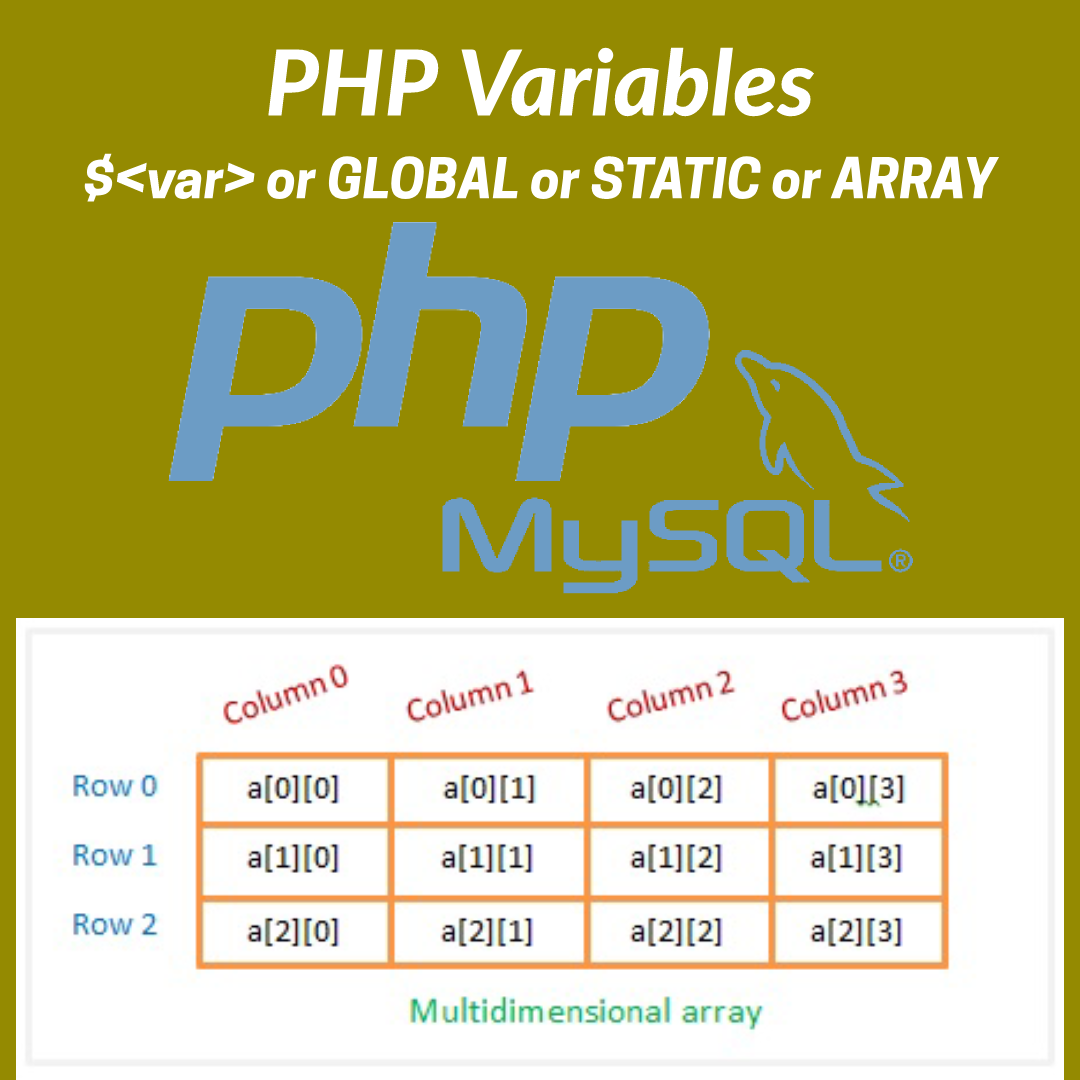# PHP variable definition and details of use global , static , and array inside or outside of ScopeTo store volatile data on a page and to use it, the variable is the most useful portion of any programming language. In this post we are going to see how to use PHP variable with its definition it contains PHP global variable, PHP array and PHP static variable with simple PHP variable and how the variable behaves inside or outside of Scope.

Generally in PHP, a variable is declared by placing \$ before the name of the variable. There is no separation of int or float etc, in case of PHP only place a dollar ( \$ ) before the variable name is done.

An example of a PHP variable is given below:

``````<?php
\$name = “Name Goes Here”;
echo \$name;
?>``````

### How to write a PHP variable and whats the structure to follow?

As already told to write a PHP variable name place \$ (dollar) before the variable name without any space and after the variable name place an equal ( = ) and then assign the value on it.

Remember the restriction to write a PHP variable.

* do not give any space between the \$ and variable name.
* do not use any special character except _ (underscore).
* should not use a _ before the variable name as its mainly used in PHP predefined key variable.

### How PHP variable behaves inside or outside of Scope?

In a simple word if a variable is declared outside of scope will nor work inside scope and similarly, if a variable is declared inside of scope will not work outside of the scope if its not a global or static variable.

Explanation with an example:

If a variable is declared first and then a function is declared, in that case, if the user wants to use the variable it will throw an error. but after the end of the function, the variable can be used again.

``````<?php
\$name = “My Name”;

function callName(){
echo \$name; //will generate an error
}

callName();

echo \$name; //will Print ‘My name’
?>``````

Similarly, if a function is declared first and inside the function, a variable is declared, the variable will work inside the function but outside of the function, that is after ends of the function if the user wants to use the variable will returns error.

``````<?php
function callName(){
\$name = “My Name”;
echo \$name; //will Print ‘My name’
}

callName();

echo \$name; //will generate an error
?>``````

### How to declare and use the PHP global variable?

As I already told that a global variable works inside and outside of scope after declaring the variable. Now to declare a global variable, first, write GLOBAL and then giving space the variable name with prefix as \$ ( dollar ). also a global variably can be declared without the sign \$ by passing the variable name inside the array of GLOBAL.

*After declaring the PHP global variable there can be assigned any value whenever needed unlike PHP static variable.

an example of PHP global variable is given below:

``````<?php
\$a = 15;
\$b = 20;

global \$a;
GLOBAL['b'];
\$b = \$a + \$b;
}

echo \$b; //the outputs will be 35
?>``````

### How to define and use PHP Static Variable?

A normal variable can be used inside scope only, after the scope the variable and its value is garbage collected. Sometimes we have to use the value of the variable outside of the scope. so that time if a variable is declared as static then its value will not be garbage collected.

an example of the PHP static variable is given below.

``````<?php
function myStaticIncrement() {
static \$a;
\$a = 1;
echo \$a;
\$a++;
}

myStaticIncrement();
myStaticIncrement();
myStaticIncrement();
//the outputs will be
// 1
// 2
// 3
?>``````

### How to declare and use a PHP Array?

To declare a PHP array user has to write a variable name using \$ ( dollar ) as a prefix and then after equal have to pass the value with the key of the array rows inside array function. There is two types of array signed and unsigned, also according to dimension PHP array is two types and they are single dimensional and multidimensional array. We will read about PHP array in another post now here I have shown how to declare a PHP array.

An example of a PHP array is given below:

``````<?php
\$unsigned_arr = array(‘red’, ‘blue’, ‘green’); // its a unsigned array

\$signed_arr = array(‘clr1’ => ‘red’, ‘clr2’ => ‘blue’, ‘clr3’ => ‘green’);//Its a signed array

\$multy_dimensional_array = array(
‘arr_ky1’=>array(‘red’, ‘blue’, ‘green’),
‘arr_ky1’=>array(‘clr1’=>’red’, ‘clr2’=>’blue’, ‘clr3’=>’green’)
); // The array is a multi dimensional array

?>``````

In this post, we have gone through PHP variable definition and details of use, PHP global variable, PHP array, PHP static variable and how does PHP variable behave inside or outside of Scope, I hope that you got a clear idea on the topic.